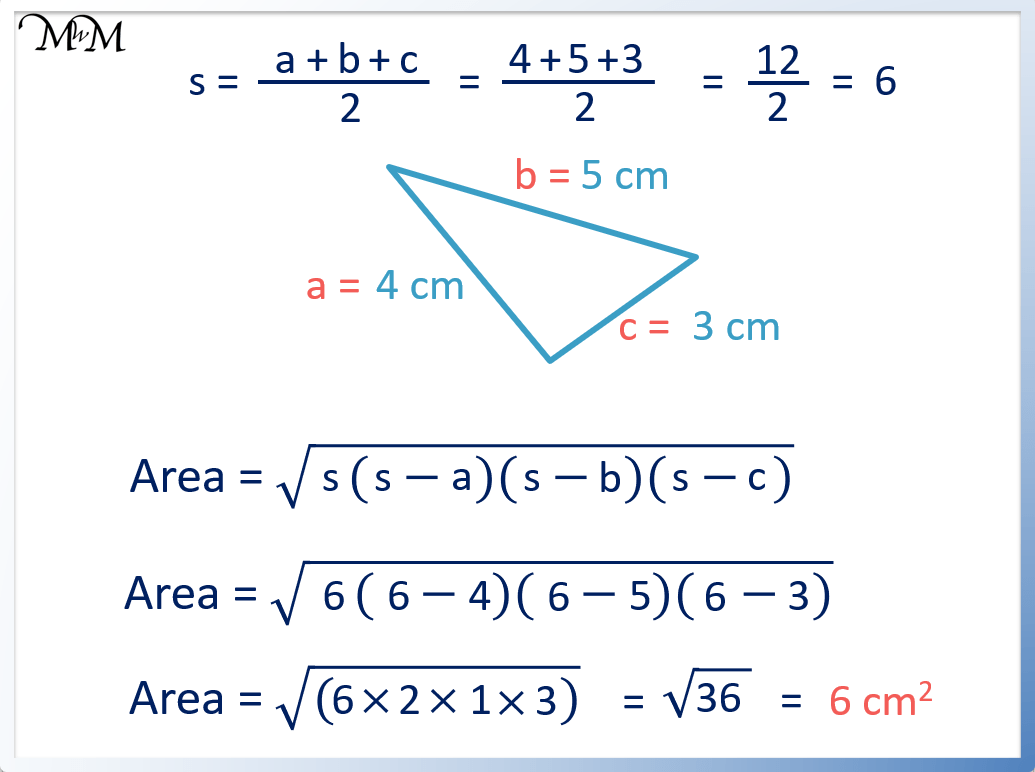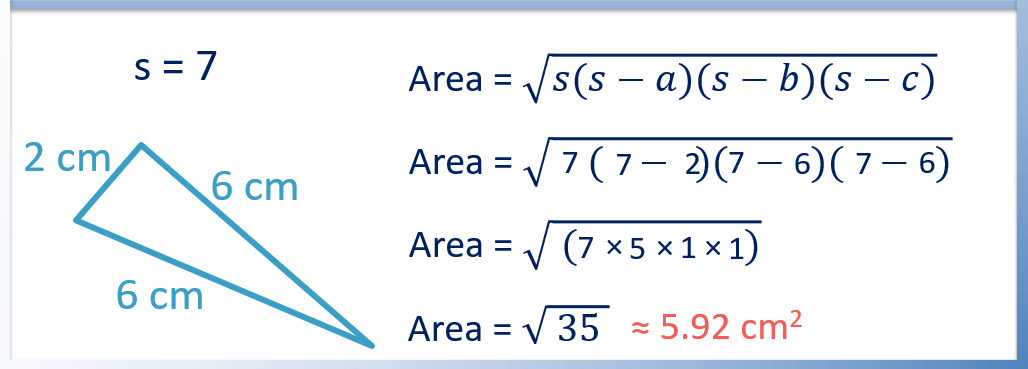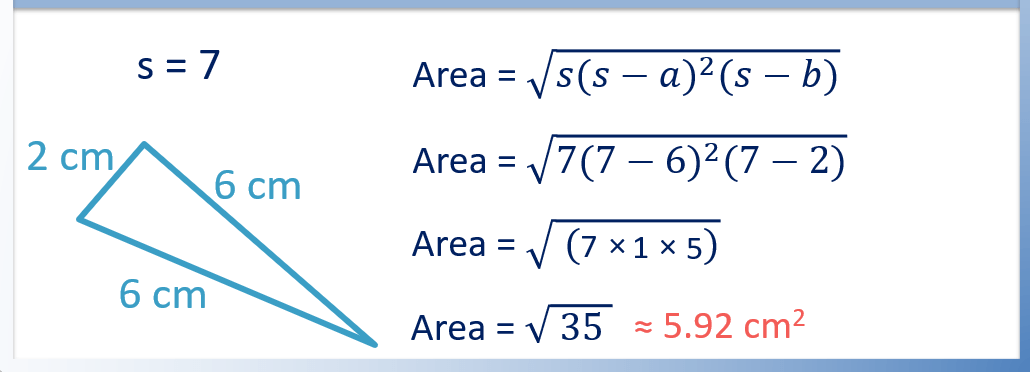# Heron’s Formula for the Area of a Triangle with 3 Sides

Heron’s Formula for the Area of a Triangle with 3 Sides• Heron’s formula to find the area of a triangle with 3 sides is $$Area = \sqrt{s(s-a)(s-b)(s-c)}$$
• a, b, and c are the side lengths of the triangle. It does not matter which side is a, b or c.
• s is the semi-perimeter, which is half of the perimeter. s = (a + b + c) ÷ 2.
• To calculate the area, we first work out s and then substitute this value along with a, b and c into Heron’s formula.

To find s, add up the sides of the triangle and divide by 2.

To find the area, substitute s, a, b and c into the formula: Area = √(s(s-a)(s-b)(s-c)).• We first label the sides a, b and c. It does not matter which side is which.
• To work out the area, we first work out s by adding up the sides and dividing by 2.
• 4 + 5 + 3 = 12 and 12 ÷ 2 = 6. s = 6.
• The area of the triangle is found by substituting s = 6, a = 4, b = 5 and c = 3 into the formula $$Area = \sqrt{s(s-a)(s-b)(s-c)}$$
• This becomes Area = √( 6(6-4)(6-5)(6-3) ), which becomes Area = √(6 × 2 × 1 ×3).
• This becomes Area = √36, which is 6.
• The area of the triangle is 6 cm2.# Area of a Triangle with 3 Sides

## How to Calculate the Area of a Triangle with 3 Known Sides

To calculate the area of a triangle with 3 known sides, use Heron’s Formula. Heron’s formula is Area = √( s(s-a)(s-b)(s-c) ), where a, b and c are the 3 side lengths of the triangle and s = (a + b + c) ÷ 2. Simply find the values of s, a, b and c and substitute these into the formula for the area.

The steps to find the area of a triangle with 3 sides (a, b and c) are:

1. Work out s = (a + b + c) ÷ 2.
2. Substitute the values of s, a, b and c into the formula of Area = √( s(s-a)(s-b)(s-c) ).

For example, find the area of a triangle with side lengths of 8 m, 3 m and 9 m.

It does not matter which sides are a, b or c.

We will set a = 8, b = 3 and c = 9.

The first step is to work out the semi-perimeter, s. The semi-perimeter is simply half of the perimeter. We find the semi-perimeter by adding up the side lengths and dividing by 2.8 + 3 + 9 = 20 and 20 ÷ 2 = 10. Therefore the semi-perimeter is 10.

s = 10.

The second step is to substitute the values of s = 10, a = 8, b = 3 and c = 9 into Heron’s Formula.Area = √( s(s-a)(s-b)(s-c) ) becomes Area = √( 10(10-8)(10-3)(10-9) ).

This becomes Area = √(10 × 2 × 7 × 1), which simplifies to Area = √140.

Finally, the square root of 140 is calculated using a calculator. Area = 11.8 m2.

## What is Heron’s Formula

Heron’s formula is Area = √( s(s-a)(s-b)(s-c) ), where a, b and c are the three side lengths of a triangle and s = (a + b + c) ÷ 2. It can be used to calculate the area of any triangle as long as all three side lengths are known. The formula is named after Heron of Alexandria (10 – 70 AD) who discovered it.

## Why use Heron’s Formula

Heron’s formula allows us to calculate the area of a triangle as long as all 3 of its sides are known. The advantage of Heron’s formula is that no other lengths or angles of the triangle need to be known. As long as the three side lengths are known, Heron’s formula works for all triangles.

## Heron’s Formula for an Isosceles Triangle

Heron’s formula for any triangle is Area = √( s(s-a)(s-b)(s-c) ). For an isosceles triangle, two sides are the same length and we can say that side c = side a. Heron’s formula for an isosceles triangle then becomes Area = √( s(s-a)2(s-b) ), where a is the length of the two equal sides, b is the length of the other side and s = (2a + b) ÷ 2.

For example, here is Heron’s formula for an isosceles triangle with side lengths of 2 cm, 6 cm and 6 cm.We can use the usual form of Heron’s formula to find the area. The semi-perimeter is the sum of the sides divided by 2.

2 + 6 + 6 = 14 and 14 ÷ 2 = 7. Therefore s = 7.

We can use Area = √s(s-a)(s-b)(s-c), which becomes Area = √7(7-2)(7-6)(7-6). This becomes Area = √35, which equals 5.92 cm2.

Here is an example of using the isosceles version of Heron’s formula: Area = √s(s-a)2(s-b). Here a = 6 and b = 2. The semi-perimeter is still 7.Area = √s(s-a)2(s-b) becomes Area = √7(7-6)2(7-2).

This becomes Area = √35, which equals 5.92 cm2. This is the same answer as before and either method can be used.

## How to Calculate the Area of a Triangle with 3 Equal Sides

The area of a triangle with 3 equal sides can be calculated with the formula Area = √3/4 a2, where a is the length of one of the sides. Alternatively, Heron’s formula for an equilateral triangle is Area = √(s(s-a)3), where a is the side length and s = 3a/2.

An equilateral triangle is a triangle with 3 equal side lengths. We will first look at finding the area of an equilateral triangle using Heron’s formula.

Heron’s formula for the area of an equilateral triangle is Area = √(s(s-a)3), where a is the side length.

In the example below, a = 8 metres.For a triangle that has 3 equal sides, the semi-perimeter is simply s = 3a/2. Here with a = 8, s = 12.

Area = √(s(s-a)3) becomes Area = √(12(12-8)3), which becomes Area = √768.

The area is 27.7 m2.

Here is another method for calculating the area of an equilateral triangle.

The formula for the area of an equilateral triangle is Area = √3/4 a2, where a is the length of one of the sides.

In this example, a = 8 metres.Area = √3/4 a2 becomes Area = √3/4 × 82.

This works out as 27.7 m2.

Both methods give the same answer.Now try our lesson on Volume of a Pyramid where we learn how to find the volume of different pyramids.error: Content is protected !!